# How to use the PROPER function in Excel

The PROPER function in excel is used to make text of Proper Case or say Title Case. The function capitalises first alphabet of each word in a cell. In this article, we will explore how you can use PROPER function.
Excel PROPER Function Syntax

=PROPER(text)

Text: it is the text or string that you want to convert in proper case. of each word in text, first latter will be capitalised.

Excel PROPER function Example 1:
In this example, user will input some names in column A. As we know first latter of each name is capital, we want the same here. But, since we can’t rely user to write in proper case, we will use excel PROPER Function. I can’t rely on me on this.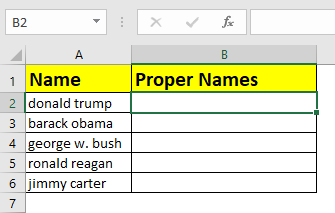Write the below formula in B1 and copy down.

=PROPER(A2)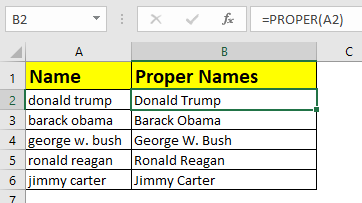Excel PROPER Example 2: Getting Only PROPER Case or Title Case Inputs
Many times you would want the user to input only proper or title case letters. In this case, we would put a data validation in excel.
Here, we have a column called verification code. Let’s say you have some guest invited in secret party. You have sent a secret code to them. They will enter their secret code in excel column. The trick is, each code should be in title case. So if any user puts a non PROPER case input, it will show error.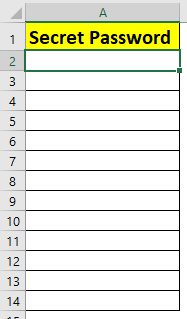1. Select first cell, Here A2.
2. Go to Data tab ? Data validation ?
3. Select Custom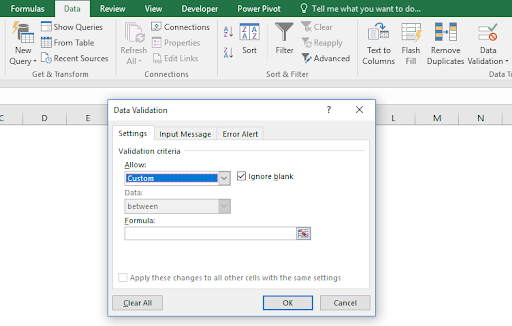1. Write this formula in formula section. Click OK. Copy this cell down.

<div="bbbb">=EXACT(A2,PROPER(A2))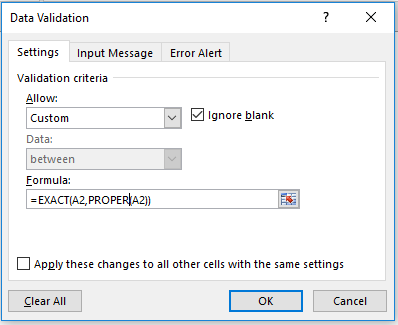Now try to enter any text that is non-proper case input.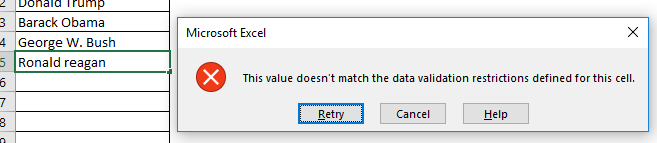It will not accept the input.
How it Works
Here, we compare text entered in cell, with PROPER case of that text using EXACT function. If they don’t match, excel shows invalid input prompt.

So yeah guys, this how you use PROPER function in excel. Let me know if you have any doubt about this function of excel in the comments section below.

Related Articles:

How to Use LOWER function in Excel

How to Use UPPER function in Excel

How to use EXACT Function in Excel

How to do Case Sensitive Lookup in Excel

Popular Articles:

50 Excel Shortcuts to Increase Your Productivity

How to use the VLOOKUP Function in Excel

How to use the COUNTIF in Excel 2016

How to use the SUMIF Function in Excel

Terms and Conditions of use

The applications/code on this site are distributed as is and without warranties or liability. In no event shall the owner of the copyrights, or the authors of the applications/code be liable for any loss of profit, any problems or any damage resulting from the use or evaluation of the applications/code.